Trance Forum | Stats | Register | Search | Parties | Advertise | Login There are 0 trance users currently browsing this page
Trance Forum » » Forum  Trance - The code for trance
 ← Prev Page 1 2 First Page Last Page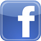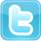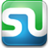Author

# The code for trance

Xolvexs
IsraTrance Senior Member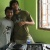Started Topics :  241
Posts :  2848
Posted : Jul 28, 2008 14:08
 8 = Infinity = Infinity ProjectWhen death comes to your doorstep, make sure you are aliveXolvexs
IsraTrance Senior MemberStarted Topics :  241
Posts :  2848
Posted : Jul 29, 2008 17:31
 theres a simplar word ARSIM...thats the code u missed the bus now wait for the assWhen death comes to your doorstep, make sure you are aliveelectrictiedye
IsraTrance Junior MemberStarted Topics :  12
Posts :  103
Posted : Jul 30, 2008 05:03
 I saw a guy wearing a jacket on which he wrote the words: "Life starts at 144BPM' loved it so I would say 144BPMSoth_MfK
IsraTrance Junior Member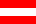Started Topics :  19
Posts :  261
Posted : Jul 30, 2008 17:38
 418 - the number of the law 93 - the number of thelemaJ
IsraTrance Full MemberStarted Topics :  193
Posts :  3858
Posted : Jul 30, 2008 19:40
 Quote: On 2008-07-30 05:03, electrictiedye wrote: I saw a guy wearing a jacket on which he wrote the words: "Life starts at 144BPM' loved it so I would say 144BPM

I'd say from 130 to 140!!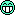IsraTrance Full MemberStarted Topics :  65
Posts :  1704
Posted : Jul 30, 2008 20:02
 a(n)=a(n-1)+a(n-2) with [a(1)=1, a(2)=1, n>2]. or (1) Polar equation: r(t) = exp(t). (2) Parameter form: x(t) = exp(t) cos(t), y(t) = exp(t) sin(t). (3) Central equation: y = x tan[ln(sqr(x˛+y˛))]. or (3) Polar equation: r(t) = at [a is constant]. From this follows (2) Parameter form: x(t) = at cos(t), y(t) = at sin(t), (1) Central equation: x˛+y˛ = a˛[arc tan (y/x)]˛.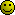episoded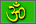Started Topics :  6
Posts :  247
Posted : Jul 30, 2008 23:40
 Quote: On 2008-07-30 20:02, Login wrote: a(n)=a(n-1)+a(n-2) with [a(1)=1, a(2)=1, n>2]. or (1) Polar equation: r(t) = exp(t). (2) Parameter form: x(t) = exp(t) cos(t), y(t) = exp(t) sin(t). (3) Central equation: y = x tan[ln(sqr(x˛+y˛))]. or (3) Polar equation: r(t) = at [a is constant]. From this follows (2) Parameter form: x(t) = at cos(t), y(t) = at sin(t), (1) Central equation: x˛+y˛ = a˛[arc tan (y/x)]˛.dude, what the hell?..see the sound
hear the colours..Braindrop
Braindrop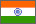Started Topics :  140
Posts :  1730
Posted : Jul 31, 2008 01:02
 303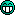www.braindrop.inJunosisStarted Topics :  2
Posts :  385
Posted : Jul 31, 2008 02:47
 how about "1212?" TB303+TR909 are essential in trance production.IsraTrance Full MemberStarted Topics :  65
Posts :  1704
Posted : Jul 31, 2008 06:44
Quote:

On 2008-07-30 23:40, episoded wrote:
 Quote: On 2008-07-30 20:02, Login wrote: a(n)=a(n-1)+a(n-2) with [a(1)=1, a(2)=1, n>2]. or (1) Polar equation: r(t) = exp(t). (2) Parameter form: x(t) = exp(t) cos(t), y(t) = exp(t) sin(t). (3) Central equation: y = x tan[ln(sqr(x˛+y˛))]. or (3) Polar equation: r(t) = at [a is constant]. From this follows (2) Parameter form: x(t) = at cos(t), y(t) = at sin(t), (1) Central equation: x˛+y˛ = a˛[arc tan (y/x)]˛.dude, what the hell?

spiralsForest dreams
IsraTrance Full MemberStarted Topics :  107
Posts :  9697
Posted : Jul 31, 2008 07:39
 forget all this.... the code is PLUR!Nothing is said that has not been said before.Xolvexs
IsraTrance Senior MemberStarted Topics :  241
Posts :  2848
Posted : Jul 31, 2008 16:52
 Plur does not sell...u cant make money with plur...plur sells only on valentines day that too for the first 20minsWhen death comes to your doorstep, make sure you are alivecorta mocas

Started Topics :  2
Posts :  145
Posted : Aug 3, 2008 05:07
 hummmm.... trance does really need to have codes?i thought It was beyond any definition....trance is trance not definition or code...........if it is worth to have any code then is LOVETrance Forum » » Forum  Trance - The code for trance
 ← Prev Page 1 2 First Page Last Page